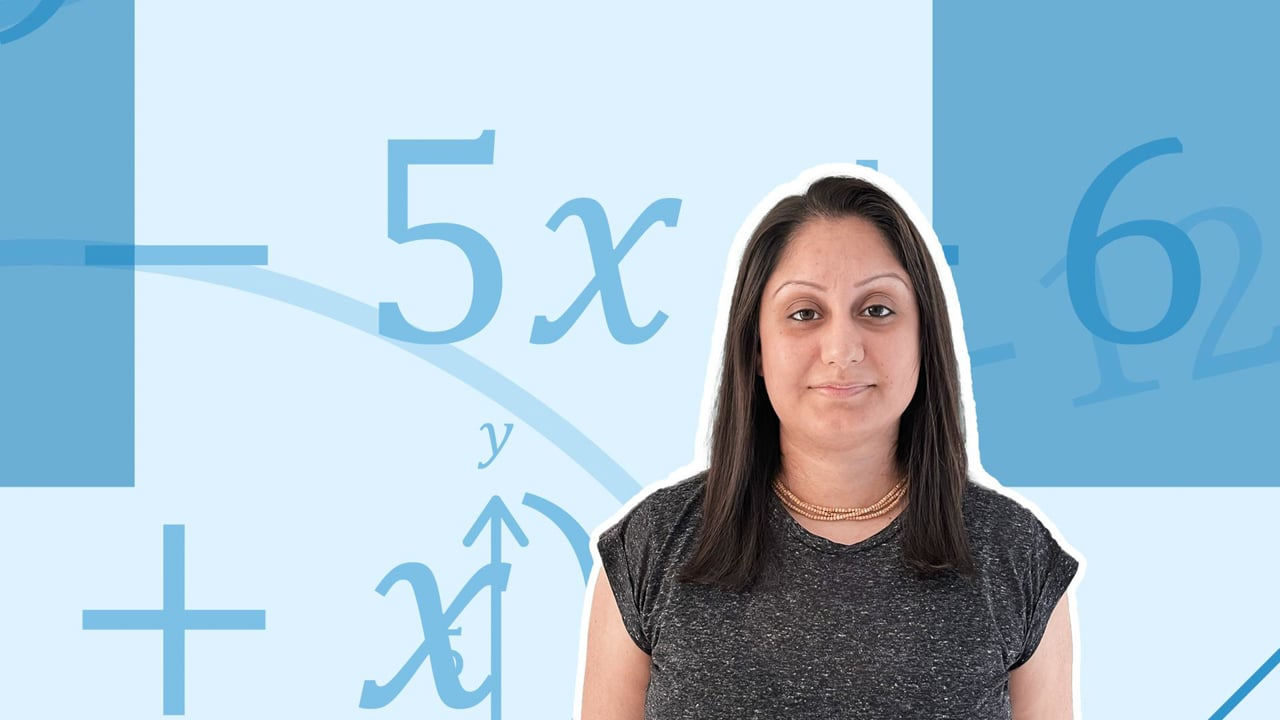Chapter overviewMaths

Types of numbers

Number calculations

Fractions, decimals and percentages

Algebraic manipulation

Formulae and equations

Straight line graphs

Other graphs

Ratio

Proportion

Rates of change

Shapes

Properties of shapes

Measures

Lines and angles

Drawing shapes

Trigonometry

Probability

Statistics

Maths

# Simultaneous equations0%

Summary

# Simultaneous equations

## In a nutshell

Simultaneous equations involves solving two (or more) equations, where the answers work in all equations given. The idea is that there is a set of solutions that work in both the equations. For two unknowns, there needs to be two​ equations to solve. Simultaneous equations can be solved using the elimination method.

#### Procedure

A) Check if the equations need to be rearranged or multiplied up for them to be written in the correct format. Write out the equations, and number them.

B) Decide whether to add or subtract equations, and solve for one of the variables. Use the rule:

 Same sign Subtract Different sign Add

C) Substitute the answer obtained into one of the equations, and solve for the other variable.

D) Check both answers in the other equation.

##### Example 1

\begin {aligned}7c+5t &=29 &\textcircled{1} \\7c+8t&= 38 &\textcircled{2} \\\underline{\qquad \quad}& \underline{\qquad \qquad \quad} \\3t&=9 &\textcircled{2} - \textcircled{1}\\t&=3 \\\\7c +5\times3 &=29 \\7c &=14 \\c&=2 \\\\7\times2 + 8\times 3 &=38 \\\underline {c=2, t=3}\end {aligned}​​

##### Example 2

\begin {aligned}7c+2d &=2 &\textcircled{1} \\-7c-5d&= 16 &\textcircled{2} \\\underline{\qquad \quad}& \underline{\qquad \qquad \quad} \\-3d&=18 &\textcircled{2} + \textcircled{1}\\d&=-6 \\\\7c +2\times-6 &=2 \\7c &=14 \\c&=2 \\\\-7\times2 -5\times -6 &=16 \\\underline {c=2, d=-6}\end {aligned}​​

##### Example 3

\begin {aligned}5p+6q&=17 \\2p+3q&=5 \qquad &\times2\\\\5p+6q&=17 \qquad &\textcircled{1} \\4p+6q&=10 \qquad &\textcircled{2} \\\underline{\qquad \quad}& \underline{\qquad \qquad \quad} \\p&=7 \qquad &\textcircled{1} - \textcircled{2}\\\\5\times7 +6q &=17 \\6q &=-18 \\q&=-3 \\\\2\times7 +3\times -3 &=5 \\\underline {p=7,q=-3}\end {aligned}

## Want to find out more? Check out these other lessons!

Solving equations

FAQs

• Question: What is the elimination method?

Answer: The elimination method means adding or subtracting the two equations to eliminate one of the variables. Only one equation should be left, with one unknown variable, so it is possible to solve the equation. This value can be used to help solve for the other variable.

• Question: What are the rules of simultaneous equations when using the elimination method?

Answer: If the signs are different, add the equations. If the signs are the same, subtract them.

• Question: What are the methods for solving simultaneous equations?

Answer: You can solve simultaneous equations using the elimination method, the substitution method, or graphically.

Theory

Exercises